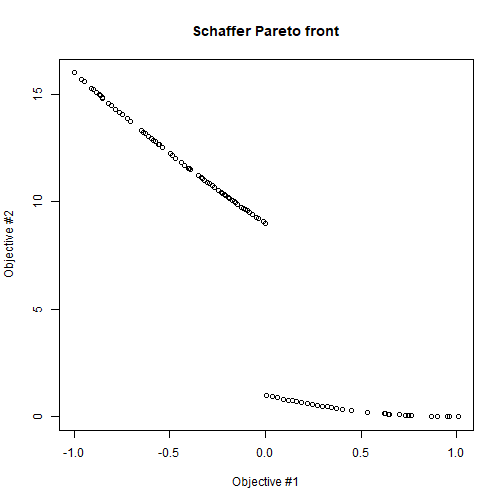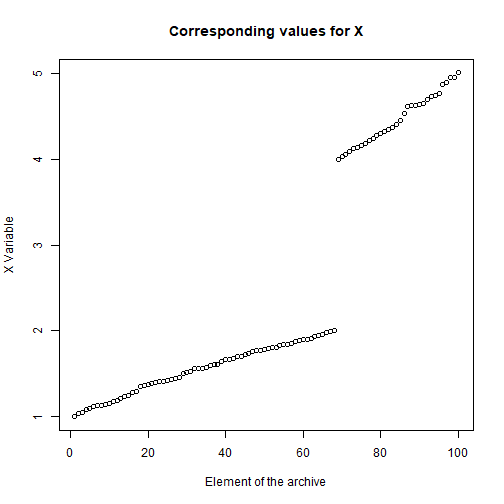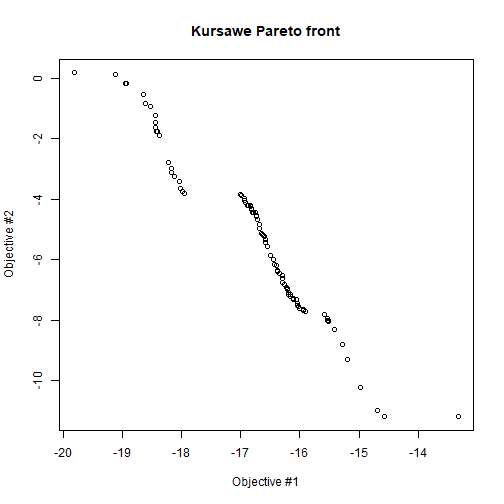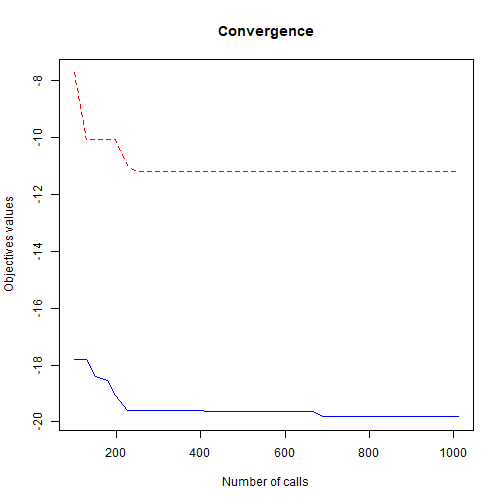# Short Description

caRamel is a multiobjective evolutionary algorithm combining the MEAS algorithm and the NGSA-II algorithm.

``````library(caRamel)
``````

# Test functions

## Schaffer

Schaffer test function has two objectives with one variable.

``````schaffer <- function(i) {
if (x[i,1] <= 1) {
s1 <- -x[i,1]
} else if (x[i,1] <= 3) {
s1 <- x[i,1] - 2
} else if (x[i,1] <= 4) {
s1 <- 4 - x[i,1]
} else {
s1 <- x[i,1] - 4
}
s2 <- (x[i,1] - 5) * (x[i,1] - 5)
return(c(s1, s2))
}
``````

Note that :

• parameter i is mandatory for the management of parallelism.
• the variable must be named x and is a matrix of size [npopulation, nvariables].

The variable lies in the range [-5, 10]:

``````nvar <- 1 # number of variables
bounds <- matrix(data = 1, nrow = nvar, ncol = 2) # upper and lower bounds
bounds[, 1] <- -5 * bounds[, 1]
bounds[, 2] <- 10 * bounds[, 2]
``````

Both functions are to be minimized:

``````nobj <- 2 # number of objectives
minmax <- c(FALSE, FALSE) # min and min
``````

Before calling caRamel in order to optimize the Schaffer's problem, some algorithmic parameters need to be set:

``````popsize <- 100 # size of the genetic population
archsize <- 100 # size of the archive for the Pareto front
maxrun <- 1000 # maximum number of calls
prec <- matrix(1.e-3, nrow = 1, ncol = nobj) # accuracy for the convergence phase
``````

Then the minimization problem can be launched:

``````results <-
caRamel(nobj,
nvar,
minmax,
bounds,
schaffer,
popsize,
archsize,
maxrun,
prec,
carallel=FALSE) # no parallelism
``````
``````## Beginning of caRamel optimization <-- Fri May 24 12:15:49 2019
``````
``````## Number of variables : 1
``````
``````## Number of functions : 2
``````
``````## Done in 4.75387096405029 secs --> Fri May 24 12:15:54 2019
``````
``````## Size of the Pareto front :
``````
``````## Number of calls : 1020
``````

Test if the convergence is successful:

``````print(results\$success==TRUE)
``````
``````##  TRUE
``````

Plot the Pareto front:

``````plot(results\$objectives[,1], results\$objectives[,2], main="Schaffer Pareto front", xlab="Objective #1", ylab="Objective #2")
````````````plot(results\$parameters, main="Corresponding values for X", xlab="Element of the archive", ylab="X Variable")
``````## Kursawe

Kursawe test function has two objectives of three variables.

``````kursawe <- function(i) {
k1 <- -10 * exp(-0.2 * sqrt(x[i,1] ^ 2 + x[i,2] ^ 2)) - 10 * exp(-0.2 * sqrt(x[i,2] ^2 + x[i,3] ^ 2))
k2 <- abs(x[i,1]) ^ 0.8 + 5 * sin(x[i,1] ^ 3) + abs(x[i,2]) ^ 0.8 + 5 * sin(x[i,2] ^3) + abs(x[i,3]) ^ 0.8 + 5 * sin(x[i,3] ^ 3)
return(c(k1, k2))
}
``````

The variables lie in the range [-5, 5]:

``````nvar <- 3 # number of variables
bounds <- matrix(data = 1, nrow = nvar, ncol = 2) # upper and lower bounds
bounds[, 1] <- -5 * bounds[, 1]
bounds[, 2] <- 5 * bounds[, 2]
``````

Both functions are to be minimized:

``````nobj <- 2 # number of objectives
minmax <- c(FALSE, FALSE) # min and min
``````

Set algorithmic parameters and launch caRamel:

``````popsize <- 100 # size of the genetic population
archsize <- 100 # size of the archive for the Pareto front
maxrun <- 1000 # maximum number of calls
prec <- matrix(1.e-3, nrow = 1, ncol = nobj) # accuracy for the convergence phase

results <-
caRamel(nobj,
nvar,
minmax,
bounds,
kursawe,
popsize,
archsize,
maxrun,
prec,
carallel=FALSE) # no parallelism
``````
``````## Beginning of caRamel optimization <-- Fri May 24 12:15:55 2019
``````
``````## Number of variables : 3
``````
``````## Number of functions : 2
``````
``````## Done in 2.61848902702332 secs --> Fri May 24 12:15:58 2019
``````
``````## Size of the Pareto front : 97
``````
``````## Number of calls : 1011
``````

Test if the convergence is successful and plot the optimal front:

``````print(results\$success==TRUE)
``````
``````##  TRUE
``````
``````plot(results\$objectives[,1], results\$objectives[,2], main="Kursawe Pareto front", xlab="Objective #1", ylab="Objective #2")
``````Finally plot the convergences of the objective functions:

``````matplot(results\$save_crit[,1],cbind(results\$save_crit[,2],results\$save_crit[,3]),type="l",col=c("blue","red"), main="Convergence", xlab="Number of calls", ylab="Objectives values")
``````# References

• Efstratiadis, A. and Koutsoyiannis, D., The multiobjective evolutionary annealing-simplex method and its application in calibrating hydrological models, EGU General Assembly 2005, Geophysical Research Abstracts, vol.7, Vienna, European Geophysical Union
• Reed, P. and Devireddy, D., Groundwater monitoring design: a case study combining epsilon-dominance archiving and automatic parameterization for the NGSA-II, Coello-Coello C editor, Applications of multiobjective evolutionary algorithms, Advances in natural computation series, vol. 1, pp. 79-100, Word Scientific, New-York, 2004